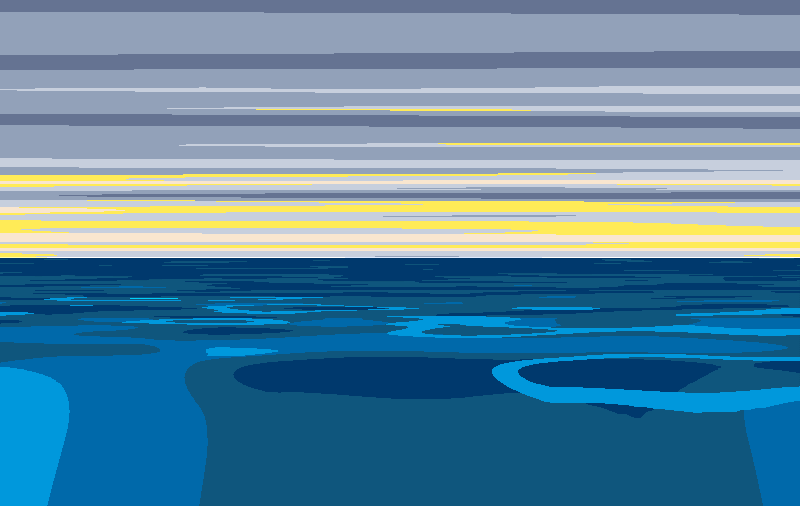How to generate pseudo-3D water with a noise texture and some color ramps

I used:

## Perspective distortion

I initally set parameters for:

• a horizon distance (how many repeats of the texture it takes to get to the horizon)
• main noise texture scale
• texture move speed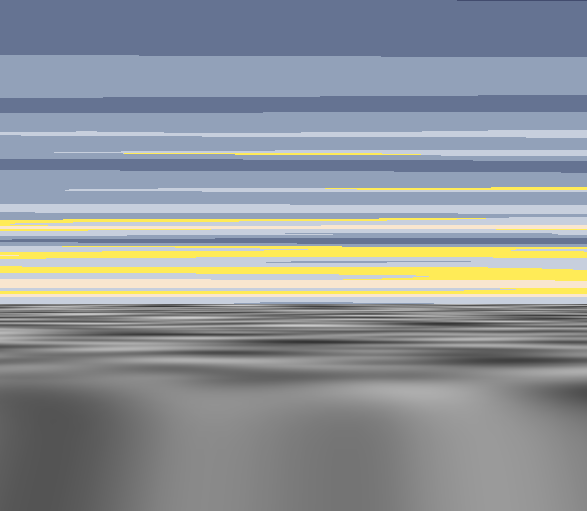// make uv x centered in screen space to put the vanishing point in the screen center
// i.e. remap from 0-1 to -1 - 1
uv.x = (uv.x*2) - 1;

// bottom of the texture should start at 0 instead of 1 for easier calculations
uv.y = 1 - uv.y;

// it needs to increase massively and then decrease a bit
float logCurve = log(uv.y)+1;
float powerCurve = pow(uv.y, 8);

// closer UVs use more of the worldPosition
uv.x = lerp(uv.x, worldpos.x, 1-logCurve);

uv.y = powerCurve * _HorizonDistance;
uv.x /= lerp(0, _HorizonDistance, 1-logCurve);

uv += _Time.x * _MoveSpeed;

fixed4 c = tex2D (_NoiseTex, uv/_MainScale.xy);
return c;


The difference between logCurve and powerCurve is important here. To mimic foreshortening as the water approaches the horizon, I use two different curves to represent distance.

For y-texture compression, I use the power curve, represented by:

$y = (x^8) * horizonDistance$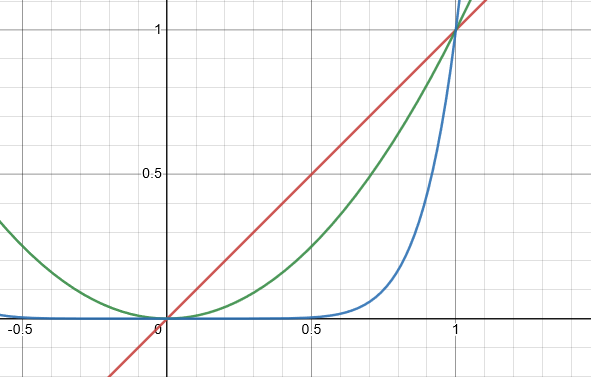Instead of the linear $$x$$ curve (red) or the $$x^2$$ curve (green), the $$x^8$$ curve (blue) gives a slow increase in computed distance that rapidly increases towards the maximum distance.

Behavior on the X axis is slightly different. To mimic parallax, the closer water needs to move with the player’s worldPos while the water further towards the horizon needs to move less, if at all. I tweaked some values and eventually settled on this distance formula for the X axis:

$y = \log_{e}(x) + 1$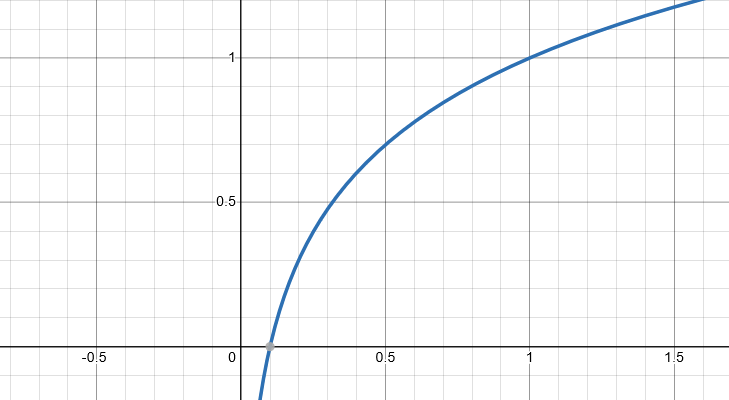## Colorization

Now that the noise was distorted correctly, I mapped it to a color. I added a new texture field for a color ramp, and provided this texture:Then I colorized the ocean by mapping lightness values and distance to the X and Y coordinates of the image.

c = tex2D(_ColorRamp, fixed2(c.r, powerCurve));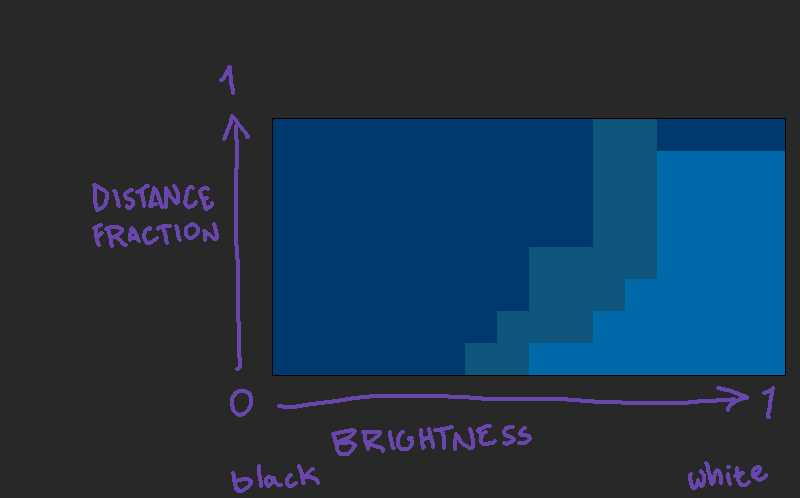With some movement, that led to this translation: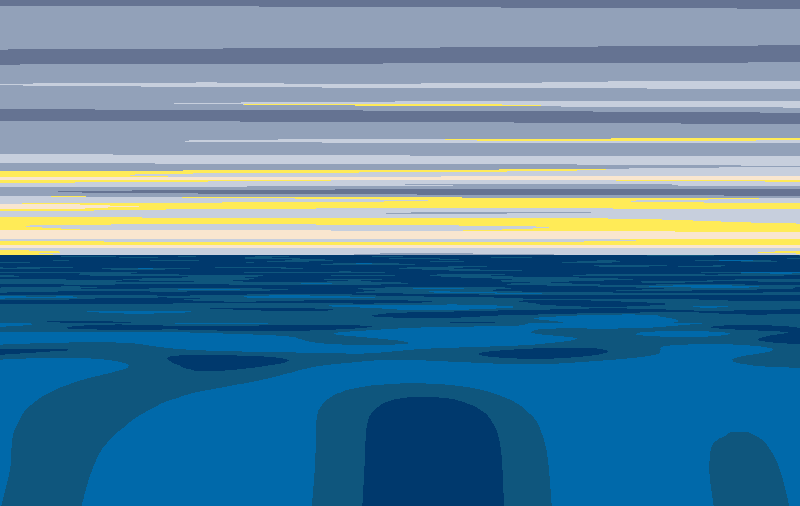Looks boring. One trick to instantly add complex movement is to overlay another texture on the first one and move it at a different rate. I added two lines of code to do that, before I do the color lookup:

	// get another color from a larger noise texture moving diagonally
fixed4 c2 = tex2D(_NoiseTex, (uv+ fixed2(_Time.x/2, _Time.z/2))/_MainScale.xy/4 );
// combine the colors of the two based on how light the second one is
c = lerp(c, c2, c2.r*0.5);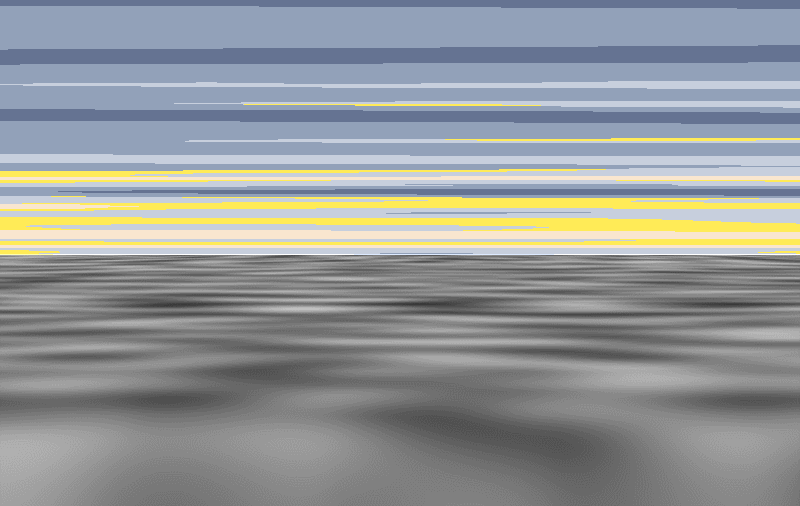This is what the noise texture looks like now before I do the color lookup.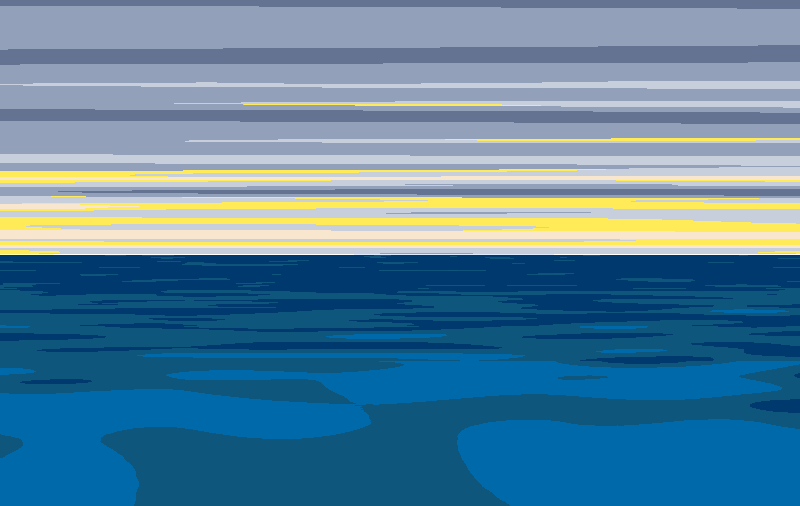Colorized, it looks much more natural and chaotic.

## Extras

I added foam moving over the surface, which was as simple as duplicating the material with a different movement speed and providing this color ramp instead: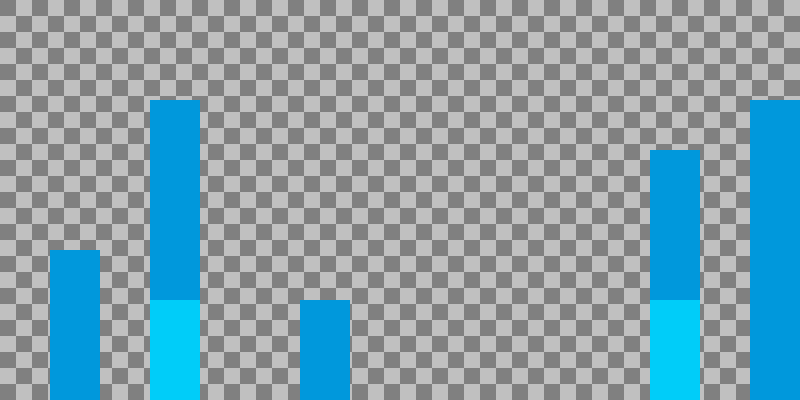That translated into the final version: